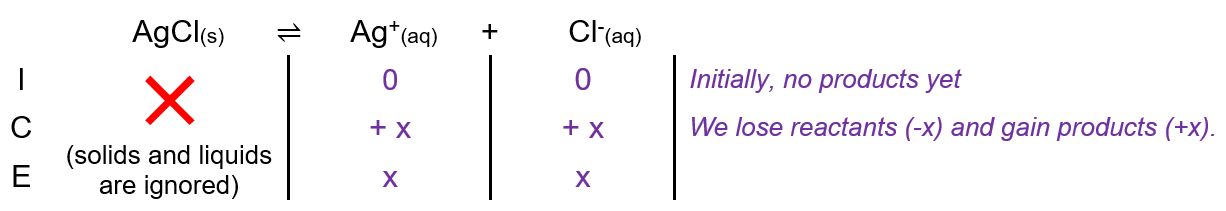# Problem: Calculate the molar solubility of AgCl (K sp = 1.8 x 10 -10 at 25 degrees C) in pure waterAgCl(s) ⇌ Ag+(aq) + Cl -(aq)

###### FREE Expert Solution

For this problem, we’re being asked to calculate the molar solubility (in mol/L) of AgCl(s) in pure water. Since the AgCl ionic, it will form ions when dissociating in water. The dissociation of AgCl in water is as follows:

AgCl(s)  Ag+(aq) + Cl(aq)

We can construct an ICE table for the dissociation of AgCl. Remember that solids are ignored in the ICE table.94% (332 ratings)###### Problem Details

Calculate the molar solubility of AgCl (K sp = 1.8 x 10 -10 at 25 degrees C) in pure water

AgCl(s) ⇌ Ag+(aq) + Cl -(aq)